Next: Switch Up: Building Blocks Previous: Gaussian variable with nonlinearity

## Discrete variables

The prior probabilities of discrete variables with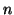possible values can be assigned fromcontinuous valued signals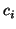using soft-max prior: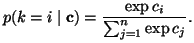(10)

The term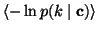of the cost function cannot be computed exactly but it can be approximated from above by using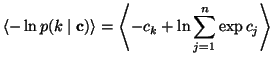(11)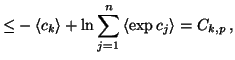(12)

which follows from the Jensens' inequality assuming all the inputs independent. Note that the terms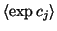appear inside the concave logarithmic function. A linear approximation based on the derivative w.r.t.therefore yields an upper bound for the cost function.

For latent discrete variables there are no restrictions to the posterior approximation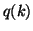. The term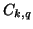in the cost function arising from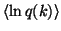is simply the negative entropy of.

The update ofis analogous to Gaussian variables: the gradient of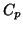w.r.t. the vector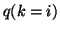with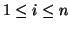is assumed to arise from a linear term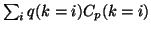, where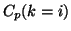denotes the value ofassuming that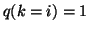. The linearity assumption holds exactly if the value of the discrete node propagates only to Gaussian variables (through switches) and corresponds to an upper bound of the cost function if the values are used by other discrete variables with soft-max prior. It can be shown that at the minimum of the cost function it holds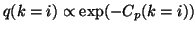.Next: Switch Up: Building Blocks Previous: Gaussian variable with nonlinearity
Harri Valpola 2001-10-01